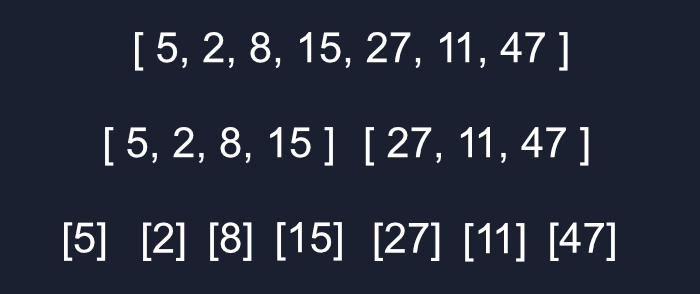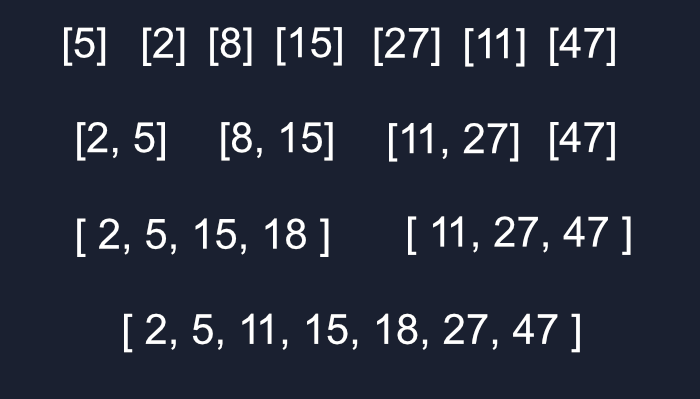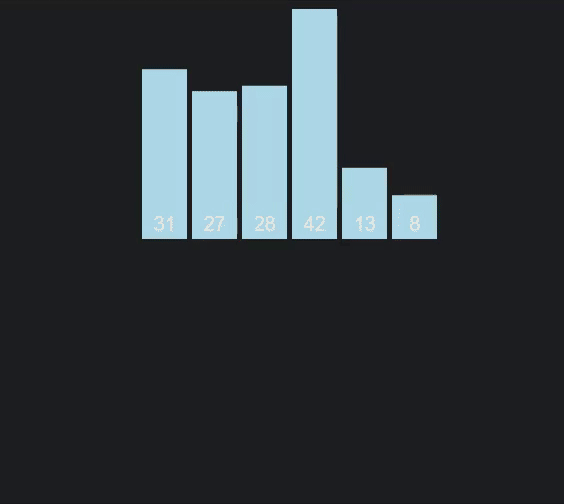# Understanding Merge Sort Through JavaScript

Published on February 8, 2020By Joshua HallWhile we believe that this content benefits our community, we have not yet thoroughly reviewed it. If you have any suggestions for improvements, please let us know by clicking the “report an issue“ button at the bottom of the tutorial.

Previously, we covered some of the beginner sorting algorithms that are able to get the job done but quickly go out of hand with larger datasets. Now we get can start digging into some of the more efficient big boy algorithms, like merge sort. With this, we can move from the `O(n^2)` algorithms to a much more scalable `O(nlogn)` solution.

## Prerequisites

Being able to think about time and space complexity via Big O Notation will be incredibly helpful. We’re also going to be looking at recursion-based examples, so you can brush up on that here.

## Concept

Similar to binary search, merge sort is a divide and conquer algorithm. The goal being to break down our problem into sub-problems and recursively continue to break those down until we have a lot of simple problems that we can easily put back together.

Merge sort is built off the idea of comparing whole arrays instead of individual items. First, we need to take the entire array and break it into many sub-arrays by continuously splitting everything in half until everything is alone in its own array. Since the amount of sub-arrays will be some multiple of the number of items in our main array, that’s what gives us the log in `O(nlogn)`.From there we can start merging, since both arrays should already be sorted we can easily compare which numbers in each is smaller and put them in the right place. This is where the n in `O(nlogn)` comes from.As you can see one half of the array is completed before the second half, and the first half of each before the next half (the different colors represent different arrays).Graphic/Animation thanks to VisuAlgo.net

## Practice Data

Here’s the array of 50 unsorted items I’ll be referring to.

``````const unsortedArr = [31, 27, 28, 42, 13, 8, 11, 30, 17, 41, 15, 43, 1, 36, 9, 16, 20, 35, 48, 37, 7, 26, 34, 21, 22, 6, 29, 32, 49, 10, 12, 19, 24, 38, 5, 14, 44, 40, 3, 50, 46, 25, 18, 33, 47, 4, 45, 39, 23, 2];
``````

## Merge

To simplify our problem we can start by creating a utility function that’ll merge two sorted arrays. There are many different ways of doing this but I found this the most succinct.

As long as there are items in either array, check if the first item in either array is smaller, then throw it into sorted and remove that item from the array with `shift()`. When that’s done, if there’s anything leftover, like when one array is larger, concatenate that onto the end.

So both arrays are gradually shrinking until one of them is empty with the leftovers thrown onto the end, since it’s already sorted.

``````const merge = (arr1, arr2) => {
let sorted = [];

while (arr1.length && arr2.length) {
if (arr1 < arr2) sorted.push(arr1.shift());
else sorted.push(arr2.shift());
};

return sorted.concat(arr1.slice().concat(arr2.slice()));
};

console.log(merge([2, 5, 10, 57], [9, 12, 13]));
``````

## Sorting

This is the easy part, we can use a recursive function to continuously cut our array in half, using `slice()` to save the data on either side of the center. It’ll return when we have our 1 item arrays then we’ll use our `merge` utility to start building them back into one large array, with every merge sorting them along the way.

``````const mergeSort = arr => {
if (arr.length <= 1) return arr;
let mid = Math.floor(arr.length / 2),
left = mergeSort(arr.slice(0, mid)),
right = mergeSort(arr.slice(mid));

return merge(left, right);
};

console.log(mergeSort(unsortedArr));
``````

## Conclusion

While Merge sort is the best algorithm we’ve covered so far since it’s `O(nlogn)`, it’s good to keep in mind that it works better with larger amounts of data. If you don’t know how much data you’ll need sorted consider using another algorithm, like insertion sort, when the dataset is small enough to get the best of both worlds.

Thanks for learning with the DigitalOcean Community. Check out our offerings for compute, storage, networking, and managed databases.Joshua Hall

author

#### Still looking for an answer?

Ask a questionSearch for more help

﻿

You can type !ref in this text area to quickly search our full set of tutorials, documentation & marketplace offerings and insert the link!

Be careful with this merge implementation. Using push will be less efficient if you initialize the array size since you already know the length. I ran a benchmark getting better results with this.

``````function merge(arrLeft, arrRight) {
const len1 = arrLeft.length;
const len2 = arrRight.length;
const len = len1 + len2;
const merged = Array(len);

let i = 0;
let l = 0;
let r = 0;
while(i < len) {
if(r === len2 || arrLeft[l] < arrRight[r]) {
merged[i] = arrLeft[l];
l++;
} else {
merged[i] = arrRight[r];
r++;
}
i++;
}
return merged;
}
``````

the approach below is about 17 times more efficient

``````const merge = (left, right) => {
const resArr = [];
let leftIdx = 0;
let rightIdx = 0;

while (leftIdx < left.length && rightIdx < right.length) {
left[leftIdx] < right[rightIdx]
? resArr.push(left[leftIdx++])
: resArr.push(right[rightIdx++]);
}
return [...resArr, ...left.slice(leftIdx), ...right.slice(rightIdx)];
};

const mergeSort = (arr) =>
arr.length <= 1
? arr
: merge(
mergeSort(arr.slice(0, Math.floor(arr.length / 2))),
mergeSort(arr.slice(Math.floor(arr.length / 2)))
);
``````

In the merge function, you are using arr.shift() inside the while loop. arr.shift() --> O(n) while(n times) --> O(n) Doesn’t it take O(n^2) time?

Click below to sign up and get \$200 of credit to try our products over 60 days!

### Popular Topics

##### Join the Tech Talk##### Hollie's Hub for Good

Working on improving health and education, reducing inequality, and spurring economic growth? We’d like to help.